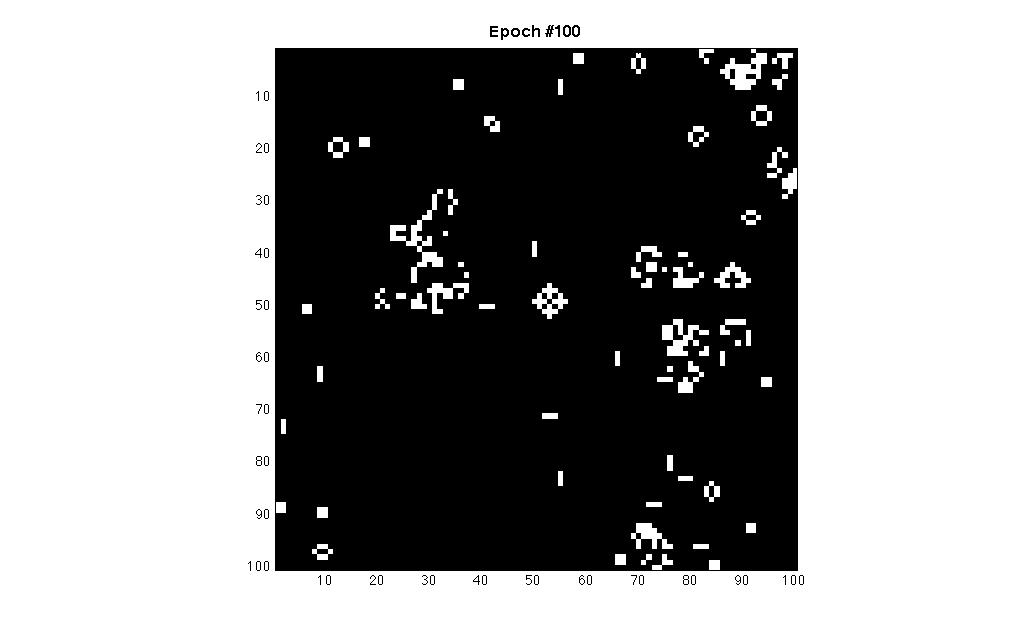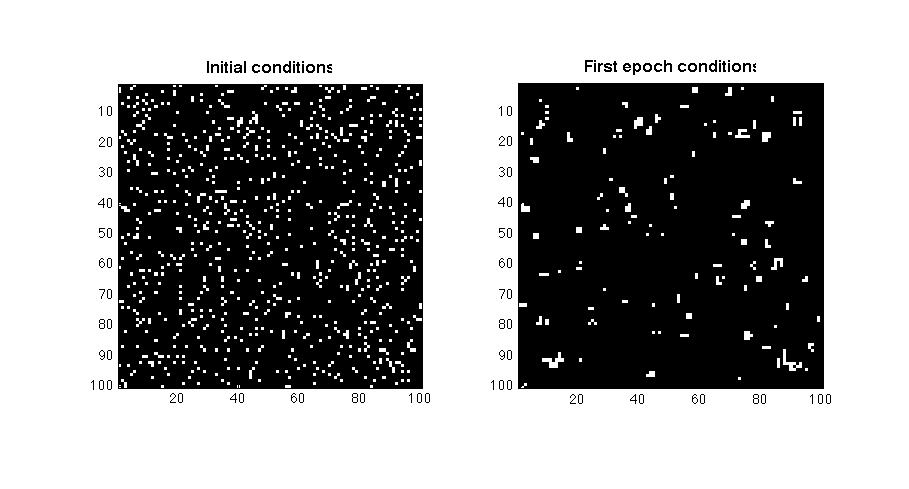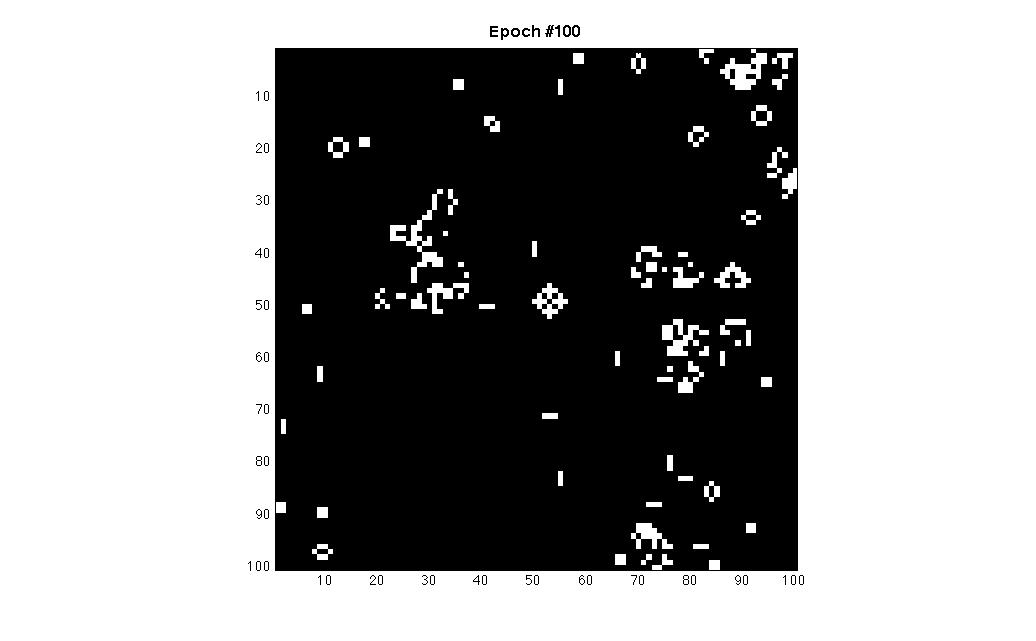The Game of Life (another MATLAB implementation)

February 16, 2014Recently I read an interesting book “The Grand Design” by Stephen Hawking and Leonard Mlodinow. Somewhere at the end of the book there was a reference to the well known game of life by John Conway (1970). Although I knew it was implemented by various researchers and is already available in MATLAB with a demo function named life, I decided to implement my own version in MATLAB using a random binary image as the initial ‘universe’ drawn by a uniform distribution of integers, provided by MATLAB function randi.
The laws of the game of life may be summarised as follows (using image processing terminology and considering the central pixel in a 3×3 pixel neighbourhood):

• Every pixel (either alive or dead) with three neighbours becomes alive in the next generation
• Every living pixel with two living neighbours makes it to the next generation
• Every other case results to the death of the pixel

So what I did was to implement a MATLAB function with the following description:

function [ MV[, INIT ] = LIFE_GAME( [BSZ[, PER[, EPOCHS[, TM[, FPS]]]]])

Executes the algorithm for the game of life as proposed by John Conway (1970)
and produces a 'movie' MV to be seen with the MATLAB function movie.

The function constructs a square binary image of size BSZ with an initial
percentage of true pixels (objects) controlled by the percentage variable PER,
then runs the game-of-life algorithm until no more changes are detected or
the number of repetitions reaches a maximum provided by the variable EPOCHS.
The initial image is produced using a variable drawn by a normal distribution.
Variables TM and FPS control the repetitions and frame-rate of the final movie
presentation in case no output arguments are defined.
The function also returns the initial binary image INIT for reference to
the initial conditions of the game.

Boundary conditions: before each iteration the image canvas is augmented by 2 pixels row
and column-wise and the image is padded with zero values

Default variable values are:
BSZ=100, PER=5, EPOCHS=200, TM=1, FPS=25

Examples:
M = life_game( 100, 12, 100);
M = life_game( 100, 17, 1000);
life_game( 100, 10, 100, 1, 25);

It should be noted that the function is far from being an optimised piece of code, as it treats all image pixels and not only the pixels needed to be taken into account. Actually a sparse matrix approach would be more adequate but the code I wrote is more easily understood.

Here is a set of images produced by the function (the initial conditions and the final situation after 100 epochs):And here is the actual MATLAB function:
The MATLAB function for running Conway’s Game of Life